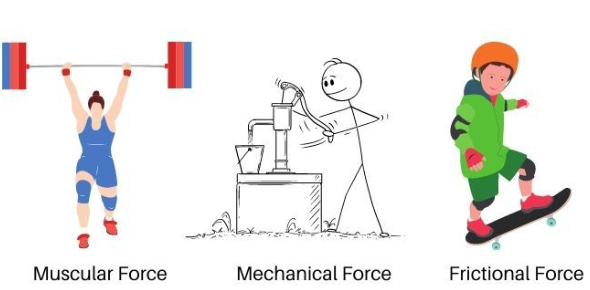# 4.2 Force And Motion Quiz

10 Questions | Total Attempts: 4063SettingsNewton's second law of motion states that a force, acting on an object, will change its velocity by changing either its speed or its direction or both. For every action there is a reaction. Have you covered the topic of force and motion extensively? Take the quiz below if you believe you have.

• 1.
The ability to do work is called-
• A.

Electricity.

• B.

Energy.

• C.

Friction.

• D.

Static.

• 2.
The result of a force moving an object through a distance is called-
• A.

Energy.

• B.

Inertia.

• C.

Work.

• D.

Static electricity.

• 3.
If an object that has energy stored in it has -
• A.

Potential energy.

• B.

Kinetic energy.

• C.

Chemical energy.

• D.

Mechanical energy.

• 4.
If an object is moving, you know it has -
• A.

Potential energy.

• B.

Kinetic energy.

• C.

Chemical energy.

• D.

Light energy.

• 5.
The terms up, down, forward, and backward are used to describe the -
• A.

Amount of energy stored in an object.

• B.

Speed of an object's motion.

• C.

Direction of an object's motion.

• D.

Amount of time an object moves.

• 6.
When a force acts on an object, the object will usually -
• A.

Stop, move, change speed, or change direction.

• B.

Melt into a liquid and move.

• C.

Create nuclear energy.

• D.

Evaporate into air.

• 7.
A moving object tends to stay in motion and a resting object tends to stay at rest -
• A.

Even if it is pushed or pulled.

• B.

Unless a force acts on it.

• C.

Until there are major weather changes.

• D.

Even if it is rolled.

• 8.
The resistance to motion caused by two objects moving against each other is -
• A.

Friction.

• B.

Inertia.

• C.

Energy.

• D.

A push.

• 9.
A push or pull on an object is -
• A.

A force.

• B.

Efficiency.

• C.

A machine.

• D.

Friction

• 10.
Heat can be created by -
• A.

Inertia.

• B.

Friction.

• C.

Efficiency.

• D.

Force.

Related TopicsBack to top